JEE  >  Test: Equation Of Plane

# Test: Equation Of Plane

Test Description

## 10 Questions MCQ Test Mathematics (Maths) Class 12 | Test: Equation Of Plane

Test: Equation Of Plane for JEE 2023 is part of Mathematics (Maths) Class 12 preparation. The Test: Equation Of Plane questions and answers have been prepared according to the JEE exam syllabus.The Test: Equation Of Plane MCQs are made for JEE 2023 Exam. Find important definitions, questions, notes, meanings, examples, exercises, MCQs and online tests for Test: Equation Of Plane below.
Solutions of Test: Equation Of Plane questions in English are available as part of our Mathematics (Maths) Class 12 for JEE & Test: Equation Of Plane solutions in Hindi for Mathematics (Maths) Class 12 course. Download more important topics, notes, lectures and mock test series for JEE Exam by signing up for free. Attempt Test: Equation Of Plane | 10 questions in 10 minutes | Mock test for JEE preparation | Free important questions MCQ to study Mathematics (Maths) Class 12 for JEE Exam | Download free PDF with solutions
 1 Crore+ students have signed up on EduRev. Have you?
Test: Equation Of Plane - Question 1

### The equation of the plane passing through the point (3, – 3, 1) and perpendicular to the line joining the points (3, 4, – 1) and (2, – 1, 5) is:​

Detailed Solution for Test: Equation Of Plane - Question 1

The equation of the plane passing through the point (3, – 3, 1) is:
a(x – 3) + b(y + 3) + c(z – 1) = 0 and the direction ratios of the line joining the points
(3, 4, – 1) and (2, – 1, 5) is 2 – 3, – 1 – 4, 5 + 1, i.e., – 1, – 5, 6.
Since the plane is perpendicular to the line whose direction ratios are – 1, – 5, 6, therefore, direction ratios of the normal to the plane is – 1, – 5, 6.
So, required equation of plane is: – 1(x – 3) – 5(y + 3) + 6(z – 1) = 0
i.e., x +  5y – 6z + 18 = 0.

Test: Equation Of Plane - Question 2

### The equation of the plane, which is at a distance of 5 unit from the origin and has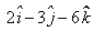as a normal vector, is:

Detailed Solution for Test: Equation Of Plane - Question 2

x = 3i - 2j - 6k
|x| = ((3)2 + (2)2 + (6)2)
|x| = (49)½
|x| = 7
x = x/|x|
= (3i - 2j - 6k)/7
The required equation of plane is r.x = d
⇒  r.(3i - 2j - 6k)/7 = 5
⇒  r.(3i - 2j - 6k) = 35

Test: Equation Of Plane - Question 3

### The Cartesian form of the equation of the plane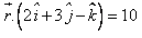is:

Detailed Solution for Test: Equation Of Plane - Question 3Let r = xi + yj + zk
(xi + yj + zk) . (2i + 3j - k) = 10
2x + 3y - k = 10

Test: Equation Of Plane - Question 4

The length of the perpendicular from the origin to the plane 3x + 2y – 6z = 21 is:​

Detailed Solution for Test: Equation Of Plane - Question 4

The given point is P(0,0,0) and the given line is 3x+2y-6z-21=0
Let d be the length of the perpendicular from P(0,0) to the line 3x+2y-6z-21=0
Then, d= |3*0 + 2*0 + (-6)*0 -21|/[(3)^2 + (2)2 + (-6)2]1/2
= |-21|/7
= 3

Test: Equation Of Plane - Question 5

The slope intercept form of the linear equation is

Test: Equation Of Plane - Question 6

If l, m, n are the direction cosines of the normal to the plane and p be the perpendicular distance of the plane from the origin, then the equation of the plane is:

Detailed Solution for Test: Equation Of Plane - Question 6

Let P(x, y, z) be any point on the plane.
OP = r = xi + yj +zk
Let l,m,n be the direction of cosine of n, then
n - li + mj + nk
As we know that r.n = d
(xi + yj + zk)(li + mj + nk) = d
i.e. lx + my + nz = d
this is the cartesian equation of plane in normal form.

Test: Equation Of Plane - Question 7

If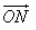is the normal from the origin to the plane, and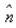is the unit vector along. P(x, y, z) be any point on the plane and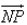is perpendicular to. Then

Detailed Solution for Test: Equation Of Plane - Question 7

NP is perpendicular to ON
NP.ON = |NP||ON| cosθ  (θ = 90deg)
|NP||ON|cos90deg
⇒ 0

Test: Equation Of Plane - Question 8

The length of the perpendicular from the origin to the plane 2x – 3y + 6z = 21 is:​

Test: Equation Of Plane - Question 9

The angle between two lines whose direction ratios are 1,2,1 and 2,-3,4 is:​

Detailed Solution for Test: Equation Of Plane - Question 9

(1,2,1) and (2, -3, 4)
Cosθ = ​(a1b1+a2b2+a3b3]/[(a1)2+(a2)2 + (a3)2)]1/2 [(b1)2 + (b2)2 + (b3)2]1/2
Cosθ = [(1)(2) + (2)(-3) + (1)(4)]/ [(1)^2 + (2)^2 + (1)2]½ [(2)2 + (-3)2 + (4)2]½
Cosθ = [2 - 6 + 4]/[1 + 4 + 1]½ [4 + 9 + 16]½
Cosθ = 0
θ = 90deg

Test: Equation Of Plane - Question 10

The equation of the plane passing through the points (2, 1, 0), (3, – 2, – 2) and (3, 1, 7) is:​

Detailed Solution for Test: Equation Of Plane - Question 10

We know that, the equation of a plane passing through three non-collinear points (x1. y1, z1), (x2 , y2 , z2) and (x3, y3, z3)
{(x-x1) (y-y1) (z-z1)} {(x2-x1) (y2-y1) (z2-z1)} {(x3-x2) (y3-y2) (z3 - z2)}
= {(x-2) (y-1) (z-0)} {(3-2) (-2-1) (-2-0)} {(3-2) (1-1) (7-0)} = 0
= {(x-2) (y-1) (z)} {(1) (-3) (-2)} {(1) (0) (7)}
=> (x-2)(-21) - (y-1)(9) + z(3) = 0
7x + 3y - z = 17

## Mathematics (Maths) Class 12

209 videos|218 docs|139 tests
Information about Test: Equation Of Plane Page
In this test you can find the Exam questions for Test: Equation Of Plane solved & explained in the simplest way possible. Besides giving Questions and answers for Test: Equation Of Plane, EduRev gives you an ample number of Online tests for practice

## Mathematics (Maths) Class 12

209 videos|218 docs|139 tests(Scan QR code)